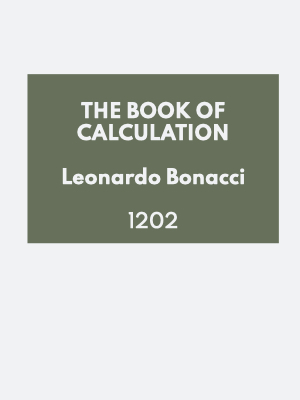# Leonardo Bonacci (fibonacci)

 Creator Name: Leonardo Bonacci (fibonacci) Creator Type: Literature / Science / Philosophy Dates: 1170 - 1250 Language: Italian

Fibonacci (; also US: , Italian:[fibonatti]; c.1170 c.1240-50), also known as Leonardo Bonacci, Leonardo of Pisa, or Leonardo Bigollo Pisano ('Leonardo the Traveller from Pisa'), was an Italian mathematician from the Republic of Pisa, considered to be "the most talented Western mathematician of the Middle Ages".

The name he is commonly called, Fibonacci, was made up in 1838 by the Franco-Italian historian Guillaume Libri and is short for filius Bonacci ('son of Bonacci'). However, even earlier in 1506 a notary of the Roman Empire Perizolo mentions Leonardo as "Lionardo Fibonacci".

Fibonacci popularized the HinduArabic numeral system in the Western world primarily through his composition in 1202 of Liber Abaci (Book of Calculation). He also introduced Europe to the sequence of Fibonacci numbers, which he used as an example in Liber Abaci.

## Biography

Fibonacci was born around 1170 to Guglielmo, an Italian merchant and customs official. Guglielmo directed a trading post in Bugia, Algeria. Fibonacci travelled with him as a young boy, and it was in Bugia where he was educated that he learned about the HinduArabic numeral system.

Fibonacci travelled around the Mediterranean coast, meeting with many merchants and learning about their systems of doing arithmetic. He soon realised the many advantages of the Hindu-Arabic system, which, unlike the Roman numerals used at the time, allowed easy calculation using a place-value system. In 1202, he completed the Liber Abaci (Book of Abacus or The Book of Calculation), which popularized HinduArabic numerals in Europe.

Fibonacci was a guest of Emperor Frederick II, who enjoyed mathematics and science. In 1240, the Republic of Pisa honored Fibonacci (referred to as Leonardo Bigollo) by granting him a salary in a decree that recognized him for the services that he had given to the city as an advisor on matters of accounting and instruction to citizens.

Fibonacci is thought to have died between 1240 and 1250, in Pisa.

## Liber Abaci

In the Liber Abaci (1202), Fibonacci introduced the so-called modus Indorum (method of the Indians), today known as the HinduArabic numeral system. The manuscript book advocated numeration with the digits 09 and place value. The book showed the practical use and value of the new Hindu-Arabic numeral system by applying the numerals to commercial bookkeeping, converting weights and measures, calculation of interest, money-changing, and other applications. The book was well-received throughout educated Europe and had a profound impact on European thought. The original 1202 manuscript is not known to exist.

In a 1228 copy of the manuscript, the first section introduces the Hindu-Arabic numeral system and compares the system with other systems, such as Roman numerals, and methods to convert the other numeral systems into Hindu-Arabic numerals. Replacing the Roman numeral system, its ancient Egyptian multiplication method, and using an abacus for calculations, with a Hindu-Arabic numeral system was an advance in making business calculations easier and faster, which assisted the growth of banking and accounting in Europe.

The second section explains the uses of Hindu-Arabic numerals in business, for example converting different currencies, and calculating profit and interest, which were important to the growing banking industry. The book also discusses irrational numbers and prime numbers.

## Fibonacci sequence

Liber Abaci posed and solved a problem involving the growth of a population of rabbits based on idealized assumptions. The solution, generation by generation, was a sequence of numbers later known as Fibonacci numbers. Although Fibonacci's Liber Abaci contains the earliest known description of the sequence outside of India, the sequence had been described by Indian mathematicians as early as the sixth century.

In the Fibonacci sequence, each number is the sum of the previous two numbers. Fibonacci omitted the "0" included today and began the sequence with 1, 1, 2, ... . He carried the calculation up to the thirteenth place, the value 233, though another manuscript carries it to the next place, the value 377. Fibonacci did not speak about the golden ratio as the limit of the ratio of consecutive numbers in this sequence.

## Legacy

In the 19th century, a statue of Fibonacci was set in Pisa. Today it is located in the western gallery of the Camposanto, historical cemetery on the Piazza dei Miracoli.

There are many mathematical concepts named after Fibonacci because of a connection to the Fibonacci numbers. Examples include the BrahmaguptaFibonacci identity, the Fibonacci search technique, and the Pisano period. Beyond mathematics, namesakes of Fibonacci include the asteroid 6765 Fibonacci and the art rock band The Fibonaccis.

## Works

• Liber Abaci (1202), a book on calculations (English translation by Laurence Sigler, 2002)
• Practica Geometriae (1220), a compendium of techniques in surveying, the measurement and partition of areas and volumes, and other topics in practical geometry (English translation by Barnabas Hughes, Springer, 2008).
• Flos (1225), solutions to problems posed by Johannes of Palermo
• Liber quadratorum ("The Book of Squares") on Diophantine equations, dedicated to Emperor Frederick II. See in particular congruum and the BrahmaguptaFibonacci identity.
• Di minor guisa (on commercial arithmetic; lost)
• Commentary on Book X of Euclid's Elements (lost)

• Fibonacci numbers in popular culture
• Republic of Pisa

### Works1202

### Movementsc. 400 - 1543

### Our Mission

The History of Creativity is a visual encyclopaedia that allows you to time travel to any time and place in the past or present.